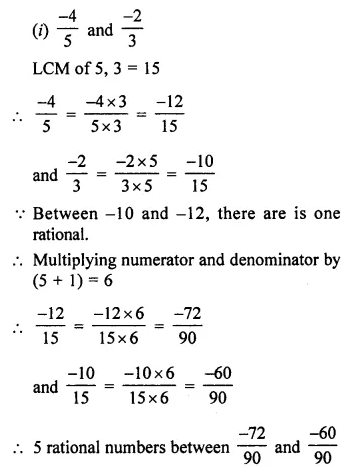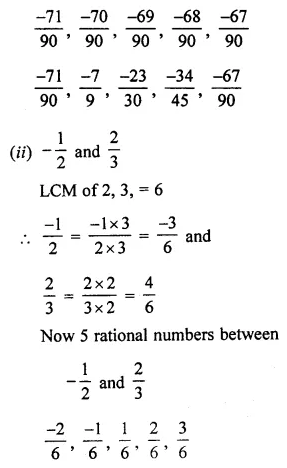# ML Aggarwal Class 7 Solutions for ICSE Maths Chapter 3 Rational Numbers Ex 3.2

## ML Aggarwal Class 7 Solutions for ICSE Maths Chapter 3 Rational Numbers Ex 3.2

Question 1.
Draw a number line and represent the following rational numbers on it:
(i) $$\frac { 3 }{ 8 }$$
(ii) $$\frac { -3 }{ 4 }$$
(iii) $$\frac { -7 }{ 8 }$$
(iv) $$\frac { -17 }{ 8 }$$
Solution: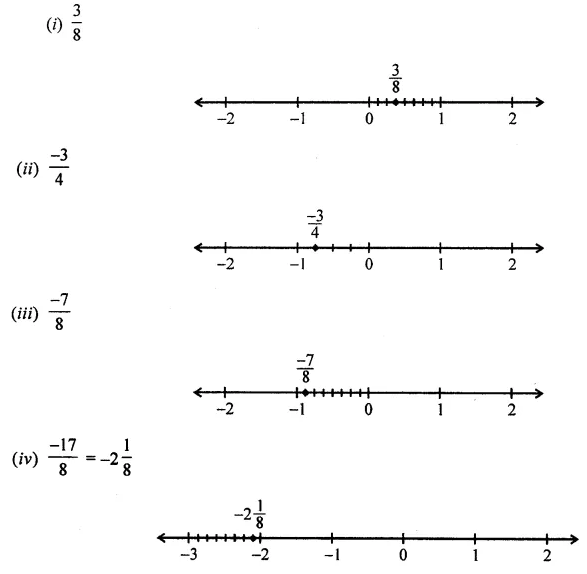Question 2.
The points P, Q, R, S, T, U, A and B on the number line are such that TR = RS = SU and AP = PQ = QB. Name the rational numbers represented by P, Q, R and S respectively.Solution: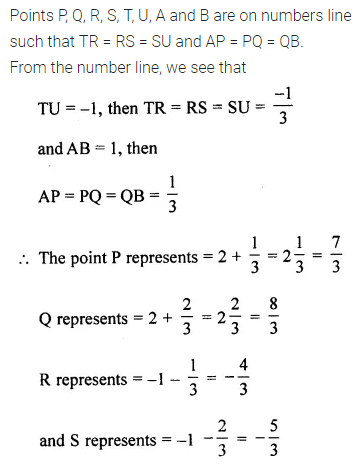Question 3.
State whether the following statements are true or false:
(i) The rational number $$\frac { -13 }{ -5 }$$ lies to the right of zeroxin the number line.
(ii) The rational numbers $$\frac { -5 }{ -7 }$$ and $$\frac { 7 }{ -9 }$$ lie on opposite sides of zero on the number line.
(iii) The rational numbers $$\frac { -17 }{ 6 }$$ and $$\frac { 8 }{ -15 }$$ lie on opposite sides of zero on the number line.
Solution: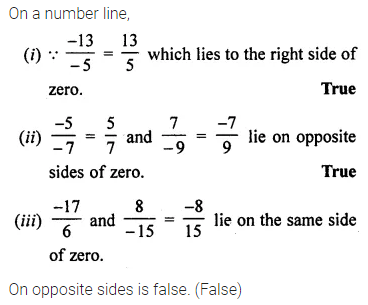Question 4.
Which of the two rational numbers is greater in each of tl following pairs?Solution: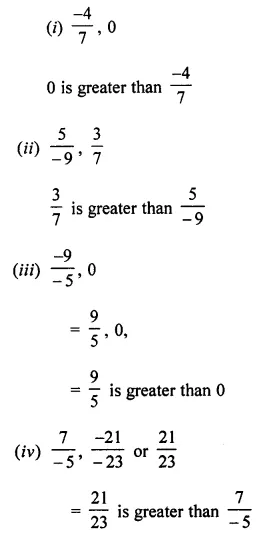Question 5.
Fill in the boxes with the correct symbol out of >, < and =:Solution: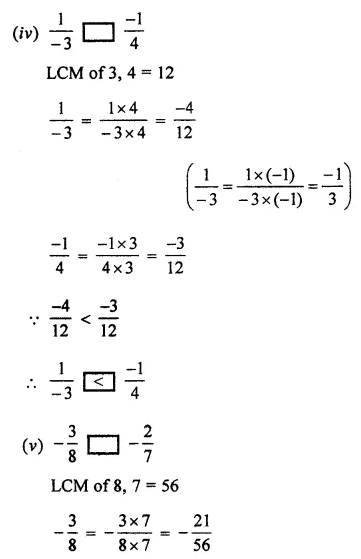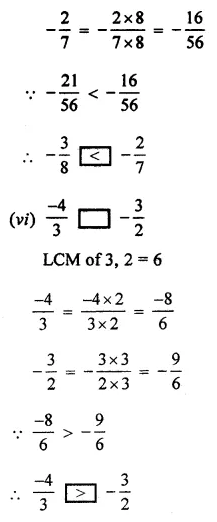Question 6.
Arrange the following rational numbers in ascending order:Solution: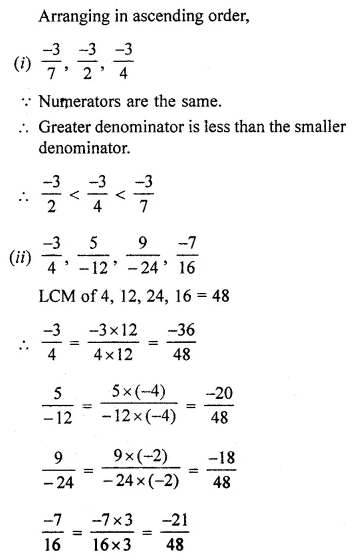Question 7.
Arrange the following rational numbers in descending order: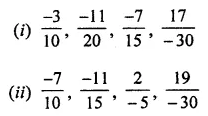Solution:Question 8.
Insert five rational numbers between:
(i) -3 and -2
(ii) $$\frac { -2 }{ 3 }$$ and $$\frac { -1 }{ 3 }$$
Solution: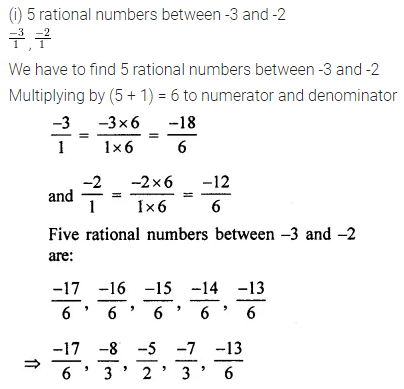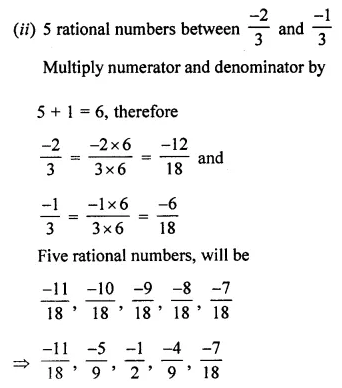Question 9.
Insert five rational numbers between:
(i) $$\frac { -4 }{ 5 }$$ and $$\frac { -2 }{ 3 }$$
(ii) $$\frac { -1 }{ 2 }$$ and $$\frac { 2 }{ 3 }$$
Solution: